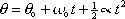theta =  theta-sub-zero    plus    omega-sub-zero times t    plus    one-half alpha times t-squared

This is one of the constant-angular-acceleration equations. It's the one with no "omega" in it.

theta [radians] is the angular position of the object at time t.
theta-sub-zero [radians] is the angular position of the object at time 0.
omega-sub-zero [radians-per-second] is the angular velocity of the object at time 0.
alpha [radians-per-seconds-squared] is the angular acceleration valid for all times.
t [seconds] is the time.

Tip: The standard units for theta are radians. In problem statements however, theta is often given in revolutions. Recognize that "the number of revolutions" is theta and convert it to radians by multiplying it by (two-pi radians over one revolution).

Pitfall Avoidance Note: Don't use any constant-angular-acceleration equation when alpha is not constant.